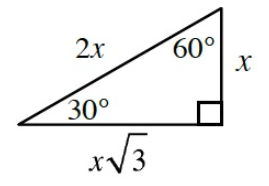### Home > INT2 > Chapter 12 > Lesson 12.2.1 > Problem12-63

12-63.

A $30°$-$60°$-$90°$ triangle has a hypotenuse of length $2$ units. Sketch and label the triangle, including the length of each side. Then use your diagram to determine exact values for the following trig expressions.1. $\text{tan }30°$

1. $\text{sin }30°$

1. $\text{cos }30°$

1. How is $\text{sin }60°$ related to $\text{cos }30°$? Is the relationship true for any pair of acute angles in a right triangle? Explain your reasoning.